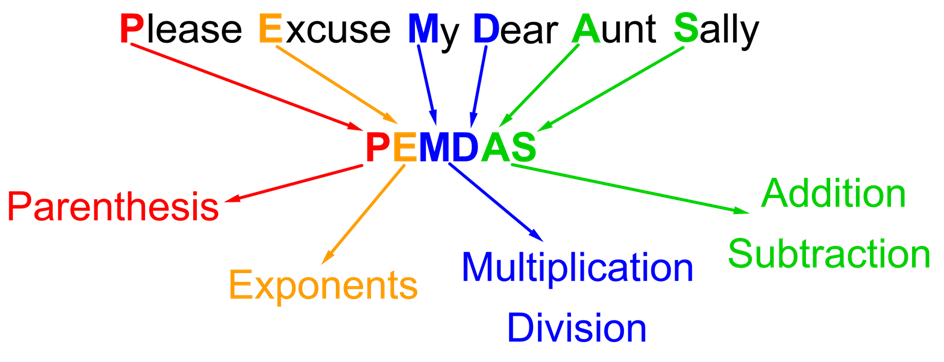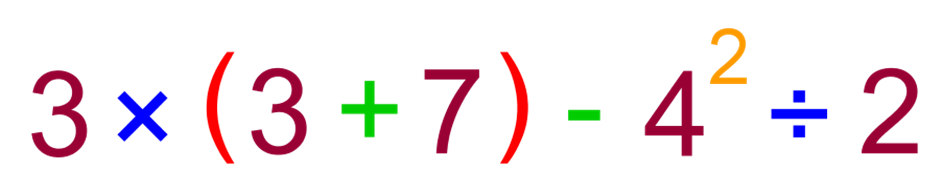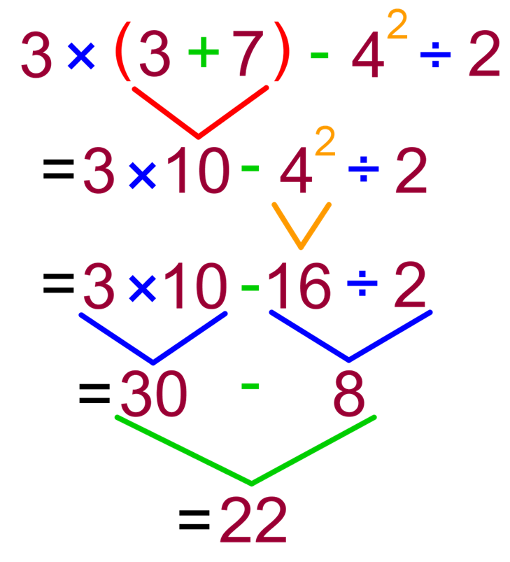# Order of operations (PEMDAS)

## What is PEMDAS?

Operations refer to adding, subtracting, multiplying and dividing in math. So what is PEMDAS and how does it relate to the order of operations?

PEMDAS is actually a memory aid to help you remember the order of which you should carry out operations. You can also use the phrase "Please Excuse My Dear Aunt Sally" to help you remember how PEMDAS is spelled. In certain parts of the world, PEMDAS is also known as BEDMAS. Let's take a deeper look at the different components of PEMDAS.

## What is the order of operations?one way to memorize PEMDAS, Please Excuse My Dear Aunt Sally

The "P" in PEMDAS stands for parentheses. It comes first in the acronym, reminding you that you should take a look at everything inside parentheses first (you may call them brackets, and therefore, some places use BEDMAS as the acronym instead).

The "E" in the acronym stands for exponents. This includes exponents and roots, so these should be tackled next. Then comes M and D, and these are a pair. "M" stands for multiply, and "D" stands for divide, but you can do either-or first. They both rank equally in the PEMDAS order. As long as you do them before "A" and "S", which stands for add and subtract. Adding and subtracting's order doesn't matter either, as long as it's all done last. Again, their rank is equal.

In general, when you're solving operations with PEMDAS, a good habit to have is to move from left to right. For example, if there's addition and subtraction in the question and the addition is more to the left, then do it before you do the subtraction.

If you calculate operations in the wrong order, you'll get the wrong answer. It's very important to pay attention and identify all the different operations you're working with so that you can correctly sort out their order. PEMDAS is actually what was agreed upon by mathematicians a long time ago in order to agree upon a consistent method to solve math problems.

## How to do order of operations

Here's an example of the PEMDAS rule being used in math. Let's try out this question:example of PEDMAS question

How do we attack this with the PEMDAS point of view? We know that the first thing we'll have to do is the parentheses. So we'll add 3 + 7, which is 10.

Now the question becomes:

$= 3 \times 10 - 4^2 \div 2$

Then comes the exponents. We have a exponent to the power of 2 on the number 4. Again, we'll solve this part of the question first before the others, giving us:

$= 3 \times 10 - 16 \div 2$

Division and multiplication are the next things we'll deal with. You can do these one by one, or just go through and do them at the same time. In this case, we'll do the $3 \times 10$, and $16 \div 2$. We now have:

$= 30 - 8$

For the last part of the question, we'll do addition and subtraction last. There aren't any additions to do, so we'll just minus 8 from 30. This gives us the final answer of 22.

A summary of the steps can be seen through this color coded image, which keep you focused on the PEMDAS rules.steps of solving the PEDMAS question

Try out this online game that can test your understanding of the order of operations. If you also wanted a place to double check your answers for PEMDAS questions, you can use this nifty math equation solver.

### Order of operations (PEMDAS)

In this section, we will evaluate operations with decimals. When evaluating operations, we will first do any calculations in brackets. Next, we will divide and multiply in order from left to right. Finally, we will add and subtract in order from left to right. In this section, we will apply our understanding of order of operations by answering given word problems.

#### Lessons

• Introduction
a)
What is PEDMAS?

b)
i) How to add decimals?
ii) How to subtract decimals?

c)
How to multiply decimals?

d)
How to divide decimals?

e)
Sign rules for Addition and Subtraction

f)
Sign rules for Multiplication and Division

• 1.
Evaluate
a)
3 + 4 × 2

b)
9 – 6 ÷ (2 + 4)

c)
8 – 3 + 2² × (7 – 6)

• 2.
This statement is missing a - and × sign. Where should the operations go to make the statement correct?
7.6 __ 5__ 9.2 = 28.8

• 3.
The Steven's purchased a 5.4 pound bag of apples at a cost of 54 cents per pound. They also bought 3 coffees at a cost of $1.50 each and 3 salads for$4 each. How much did they spend in total? Write a single expression to show how to calculate the answer. Use a calculator to find the value of the expression.

• 4.
To train for an open water swim event, Samantha swims 2 km per day on Mondays and Wednesdays and bikes 10.5km per day on Tuesdays and Thursdays. How many km in total does she swim and bike each week?